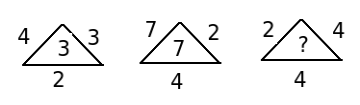# Logical reasoning - Quantitative Aptitude (MCQ) questions for Q. 21477

Q.  Find the missing number- Published on 04 May 16

a. 5
b. 8
c. 4
d. 2
e. None of these

We have :
4 * 3 * 2 / 8 = 24/8 = 3
7 * 2 * 4 / 8 = 56/8 = 7
Thus, missing number = 2 * 4 * 4/8 = 32/8 = 4

#### Discussion

• Ridish   -Posted on 30 Aug 16
Nice